Often when reading a good maths book, the author will get to the end of an explanation of a particularly complicated proof, theorem, or idea, and mention the “beauty” of the maths involved. I always wonder what, exactly, this means. Did I miss a particularly neat diagram? Or, as seems to be the case, is mathematical beauty something buried deep: something that, perhaps, I need a PhD to get to grips with?

I used to think that it was the latter — maybe one day, after years of studying maths at its highest level, I’d suddenly gain a glimpse of some incomprehensibly deep truth and realise the incredible beauty of things which now seem boring and trivial.

Maths can be like a dense jungle — it’s hard to penetrate but you never know whom you might might.

But actually, I think you can get a glimpse of what mathematicians mean by beauty without too much effort at all. That’s what I’m going to try and convince you of in the rest of this article. Mathematics can be a bit like a dense, never-ending jungle. It can feel like you’re hacking away and away at it and never getting anywhere, but if you stop and look around yourself, every once in a while you see incredible, exotic plants and animals to marvel at — and ever so often you find large new swathes of jungle to explore.

The particular thing that I want to introduce you to, that I think is so beautiful, is something that was mentioned in passing on a television programme I was watching. I hardly knew what it meant, and I certainly had no idea how it came about, but I knew I had to find out more.

I am talking about Euler’s identityNow you probably think I’m crazy. What’s beautiful about that? Well, I ought to warn you, I’m not alone — Mathematical Intelligencer readers voted the identity the “most beautiful theorem in mathematics”. The physicist Richard Feynman called the formula it is derived from “one of the most remarkable, almost astounding, formulas in all of mathematics”.

But what is so special about it? Well, first I ought to explain what the symbols actually mean.

You’re probably familiar with, it’s the ratio between a circle’s circumference and its diameter. The numberis also a constant, and you may be vaguely familiar with it as the base of the natural logarithm. To 20 decimal places,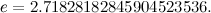Bothandare irrational numbers – they have an infinite number of decimal places and you can’t write them down as one integer divided by another.

Probably the strangest of these three numbers is. It is the square root of -1, that is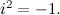It’s called an imaginary number, and you can’t find it anywhere along the normal number line, as none of the ordinary real numbers give a negative number when squared.

Are you starting to get an idea of the beauty of Euler’s identity? If you take the constantto the power of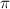multiplied by, and then take away 1, you get to 0. Isn’t it a little odd how three very strange numbers which are not connected in any evident way combine to give such a normal and familiar result?Euler’s identity is named after Leonhard Euler, one of the most prolific mathematicians of all times.

So, why does this happen? You might think that it is down to some really complex idea — how do we even take a number to the power of? Well, actually, it isn’t too difficult to see how Euler’s identity comes about – that is one thing that makes the identity so wonderful! But first you have to see Euler’s formula, which leads to his beautiful identity, in full generality:Doesn’t look quite as nice and neat now, does it? But don’t be put off. To understand how this formula comes about, we need something called Taylor series. These are just a way of expressing functions such as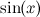or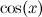as infinite sums. They were discovered by the mathematician Brook Taylor (who was also part of the committee which adjudicated the argument between Isaac Newton and Gottfried Leibniz about who first invented the calculus).

The Taylor series for the function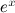is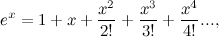wheredenotes the productYou can verify this Taylor series using a calculator: choose a numberand see what value the calculator gives you for. Now use the calculator to work out the value of the sum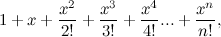for as many terms as you like, that is for a number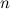as high as you like. You will find that the result very nearly equals the result you got forand the more terms you add to the sum, the closer the two results become. At some point the two results will be the same on your calculator, as their difference becomes too small for the calculator to detect. In “reality”, the two results are equal when you have added an infinite number of terms to your sum.

The Taylor series for the other two functions appearing in Euler’s formular are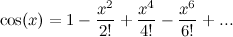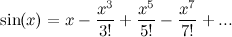Again you can check this using your calculator, bearing in mind that the angle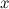is measured in radians, rather than degrees.

Now let’s multiply the variablein the Taylor series forby the number. We getBut certain powers ofcan be simplified – for example,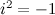by definition, and so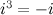and, and so on. So we can simplify the above toWe can gather the terms involvingtogether to give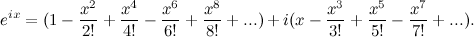Now notice that these two series are the same as the series forandfrom earlier, so we can substitute these in to get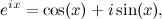which is Euler’s formula!

All we have to do now is substitute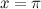. Sinceandwe get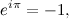soSo you see, after a sequence of fairly complex mathematics we arrive back where we started — at the (seemingly) simple numbers 1 and 0. That is what I think is so beautiful about this identity: it links very strange numbers with very ordinary and fundamental ones. Seeing why it works feels a bit like treading a little-known path through the mathematical jungle to reach a secret destination somewhere in the thick undergrowth.